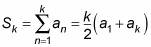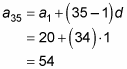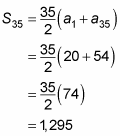##### Pre-Calculus All-in-One For DummiesWhen your pre-calculus teacher asks you to calculate the kth partial sum of an arithmetic sequence, you need to add the first k terms. This may take a while, especially if k is large. Fortunately, you can use a formula instead of plugging in each of the values for n. The kth partial sum of an arithmetic series isYou simply plug the lower and upper limits into the formula for an to find a1 and ak.

Arithmetic sequences are very helpful to identify because the formula for the nth term of an arithmetic sequence is always the same:

an = a1 + (n – 1)d

where a1 is the first term and d is the common difference.

One real-world application of an arithmetic sum involves stadium seating. Say, for example, a stadium has 35 rows of seats; there are 20 seats in the first row, 21 seats in the second row, 22 seats in the third row, and so on. How many seats do all 35 rows contain? Follow these steps to find out:

1. Find the first term of the sequence.

The first term of this sequence (or the number of seats in the first row) is given: 20.

2. Find the kth term of the sequence.

Because the stadium has 35 rows, find a35. Use the formula for the nth term of an arithmetic sequence. The first term is 20, and each row has one more seat than the row before it, so d = 1. Plug these values into the formula:Note: This solution is the number of seats in the 35th row, not the answer to how many seats the stadium contains.

3. Use the formula for the kth partial sum of an arithmetic sequence to find the sum.